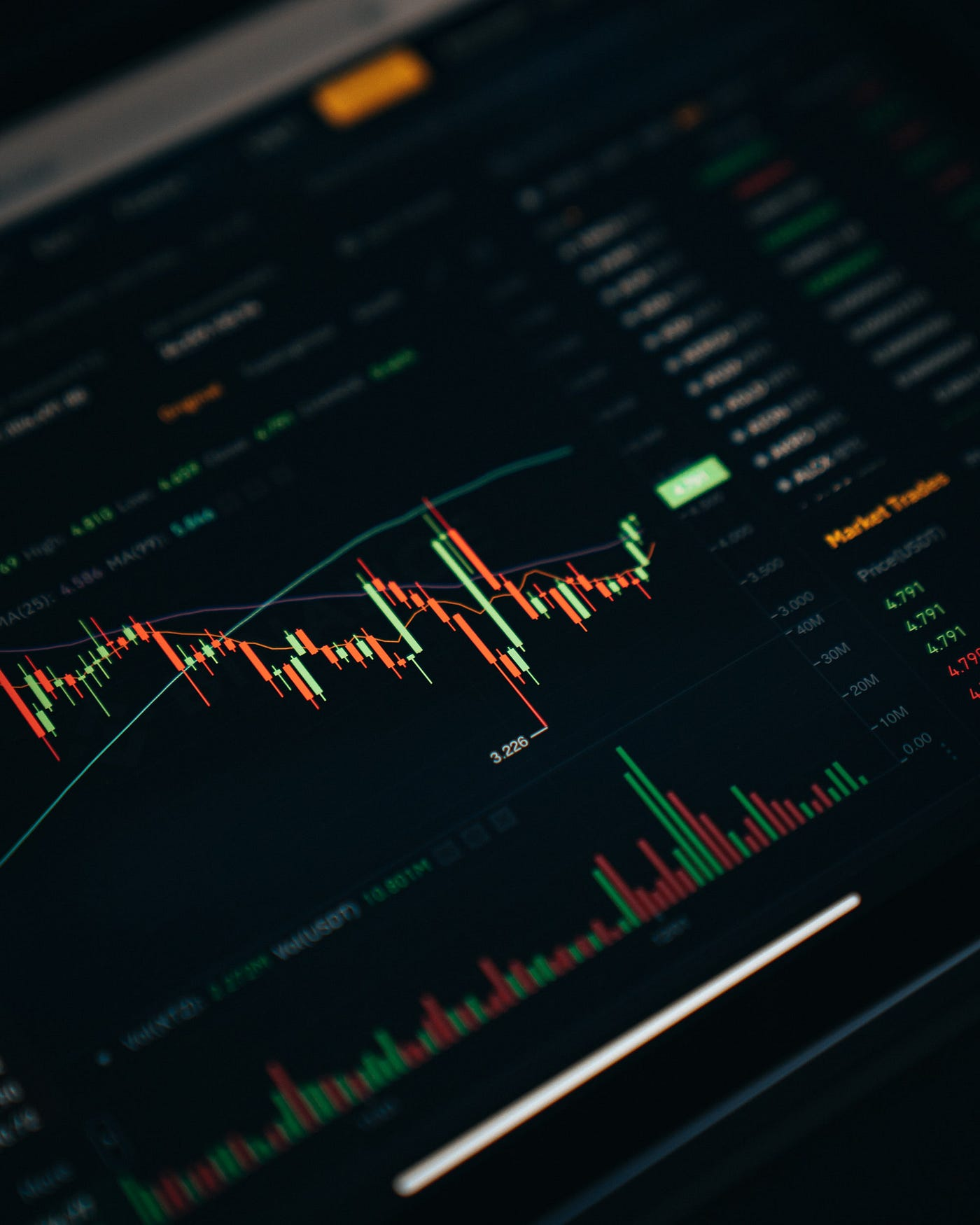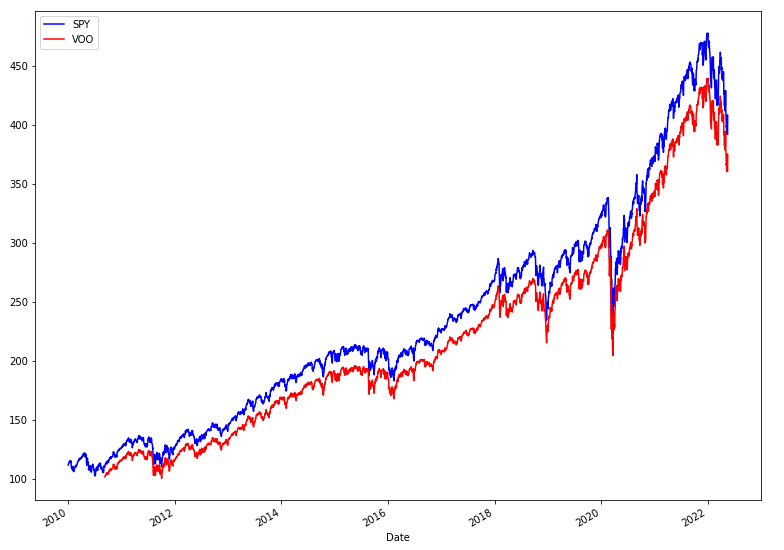# Five useful Pandas scripts for financial time series plots

##### Share:

In one of my recent posts, I’ve made public a set of useful scripts for financial series. Having those numbers in place is just half of a job, so I’m glad to continue community contribution by sharing another portion today. This time is dedicated to visualising information.Photo by Dylan Calluy on Unsplash

# Candlestick

How to use:

`plot_candlestick(df)plt.xlim([datetime.date(2022, 1, 1), datetime.date.today()])`

Candlestick plots show the opening and closing prices for a given period and the prices of high and low. You can use this information to identify patterns and predict future price movements. For example, a bullish candlestick plot indicates that buyers are in control, and prices will likely rise. On the other hand, Bearish candlesticks suggest that sellers are in the driver’s seat, and prices could fall. While candlestick plots can’t tell you definitively where prices are headed, they can be a helpful tool for analysis.

# Fibonacci Retracement

How to use:

`plot_candlestick(df)plot_fibonacci_retracement(df)`

Fibonacci Retracement plots are technical analysis tools used to identify potential support and resistance levels for given security. The Fibonacci Retracement is based on the Fibonacci sequence, which is a series of numbers in which each subsequent number is the sum of the previous two. The most common Fibonacci Retracement levels are 23.6%, 38.2%, 50%, 61.8% and 78.6%. These levels are derived from the Fibonacci sequence and indicate areas where the security’s price may find support or resistance. Technical analysts will often use Fibonacci Retracement plots in conjunction with other technical indicators to confirm potential support and resistance levels.

# Benchmark

How to use:

`spy = yf.download(f’SPY’, start=’2010–01–01')voo = yf.download(f’VOO’, start=’2010–01–01')spy.rename(columns = {‘Close’: ‘SPY’}, inplace = True)voo.rename(columns = {‘Close’: ‘VOO’}, inplace = True)plot_against_benchmark(spy[‘SPY’], voo[‘VOO’])`Two line plots against each other with a single Y-axis

While this type of plot is quite simple, I’m using it in almost every analysis because of its practical use case — comparing it with some basic expectations is an excellent place to start. One good example is to plot any stock against S&P500 or NASDAQ.

Note: Try using the code above with two_axis = True parameter

# Volume

How to use:

`import datetimeprice_plot = plt.subplot2grid((5,4), (0, 0), rowspan=3, colspan=4)plot_candlestick(df, ax = price_plot)volume_plt = plt.subplot2grid((5,4), (3,0), rowspan=1, colspan=4, sharex = price_plot)plot_volume(df, aggregation = ‘daily’, ax = volume_plt)plt.gcf().set_size_inches(13, 10)plt.figure()`

When looking at a volume plot, there are a few things to consider. First, the volume plot can help you identify sharp increases or decreases in trading activity. This can be useful in spotting potential trends. Second, the volume plot can also give you an idea of interest in a particular commodity. It is also important to remember that volume is just one factor to consider when making investment decisions. In addition to looking at the volume plot, you will also want to look at other factors such as price movement and news sentiment.

# Exponential Moving Average

How to use

`candle = plot_candlestick(df)plot_ewm(df, ‘Close’, 14, ax = candle.gca())`

Exponential moving average plots can examine trends in data, identify outliers, and predict future values. When creating exponential moving average plots, it is important to use an appropriate number of data points so that the plot is representative of the underlying data set.

# Request to Readers

Recently I’ve posted a few successful (in terms of this blog) articles about time series and how to plot them, hence the question:

Would you like to have a service with tools for analysing and rendering time series?

The entire code for the article and a bit more was pushed to the GitHub repository:

Similar posts:

No Free Lunch